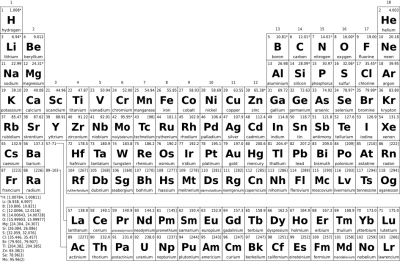# Choose one of the following elements (C, H, O, N), and answer questions 1-6 on it: - Atomic...

## Question:

Choose one of the following elements (C, H, O, N), and answer questions 1-6 on it:

- Atomic Number

- Mass Number

- Number of electrons in the outer shell

- Number of protons

- Number of electrons

- Number of neutrons

## Common Elements In Living Things:

The most common elements found in living things are C, H, O, N, P and S. These are all in the top two rows of the periodic table, and thus they are among the lightest elements. Together, they form the backbone of the common organic molecules found in living things, proteins, carbohydrates, lipids, and nucleic acids.

Below is a table that summarizes all six items from the question prompt for each of the following atoms: C, H, O, N, P, and S. First let's see how each of these is obtained. The atomic number for each atom is directly on the periodic chart, it is always an integer. The mass number is found from the atomic mass found on the periodic chart. The atomic mass found on a periodic chart is an average mass including all the isotopes of a particular atom. The mass number for the typical atom of a particular element is found by rounding the atomic mass. For example, the mass number of nitrogen is 14, for oxygen it is 16. An isotope of an atom would have a different mass number as an isotope has a different number of neutrons than the typical atom of that element. For example, most carbon has an a mass number of 12. But C-14 has a mass number of 14 since it has two extra neutrons.

The number of protons is always equal to the atomic number. The number of neutrons is equal to the mass number minus the atomic number. Unless an atom is an ion, the number of electrons is equal to the number of protons, or the atomic number. The electrons in the outer shell is shown directly on some periodic charts. For the first 20 atoms, which includes many of the atoms found in living things, which column an atom is in on the periodic chart tells us how many electrons are in the outer shell. For example, H is in the first column and has 1 electron in the outer shell. C is in the 4th column, and it has 4 electrons in the outer shell.

Property How Determined C H O N P S
Atomic Number on the periodic chart 6 1 8 7 15 16
Mass Number equals the mass on the periodic chart, rounded 12 1 16 14 31 32
Protons equals the atomic number 6 1 8 7 15 16
Neutrons equals mass number minus atomic number 6 0 8 7 16 16
Electrons equals atomic number in neutral atoms 6 1 8 7 15 16
Electrons in Outer Shell same as column number for atoms with atomic number 1-20 4 1 6 5 5 6